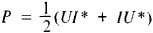# Complex Amplitude

The following article is from The Great Soviet Encyclopedia (1979). It might be outdated or ideologically biased.

## Complex Amplitude

a representation of the amplitude A and phase Ψ of a harmonic oscillation x = A cos (ωt + Ψ) with the aid of the complex number Ã = A exp(iΨ) = A cos Ψ + iA sin Ψ. In this case, the harmonic oscillation can be described by the expression x = Re [Ã exp(iωt)], where Re is the real part of the complex number within the square brackets.

Complex amplitudes are usually used in the design of linear electric networks (with a linear dependence of current on voltage) containing resistive and reactive elements. If a harmonic electromotive force (emf) of frequency ω acts on such a circuit, then the use of complex amplitudes for the current and voltage makes possible the use of algebraic equations instead of differential equations. The relationship between the complex amplitudes of the current I and voltage U for a resistance R is determined by Ohm’s law, I = U/R. For an inductance L this relationship has the form I = U/iωL, and for a capacitance C, the form I = iωCU. Thus, the quantities iω L and 1/iωC play the roles of inductive and capacitive reactances.

The computation of the complex amplitude of the current for a section of an electric network containing the elements L, C, and R on which an external harmonic emf of frequency ω is acting is carried out with the aid of a relationship analogous to Ohm’s law: I = Ũ/Z(ω). Here Z is the impedance of the given section of the network and may be found by means of the same rules as series and parallel arrangements of resistances in a DC network. The complex amplitude of the current found in this way allows the determination of the amplitude and phase of the actual current flowing through the circuit.

The method of complex amplitudes can be applied to any periodic influence on a linear network. In this case, the external nonharmonic influence must be expanded into a Fourier series, after which the computation is carried out for each of the harmonic components of the external influence and the results obtained are summed. In the calculation by the complex-amplitude method of the mean power P = 1/2IU cos Φ, where Φ is the phase shift between the current and the voltage, it is necessary to use the rule that the actual power is equal toHere I* and U* are the complex conjugates of the amplitudes of the current and voltage.

V. N. PARYGIN

References in periodicals archive ?
Instead, we model it by using an impulse-like, bell-shaped functions, 200 days long and centered at GMJ epochs [t.sub.0], whose complex amplitude a is estimated to obtain the best fit to observations:
where g(x, y) and G([xi], [eta]) denote the complex amplitude in the spatial domain and Fourier domain, respectively, [absolute value of g(x, y)] and [absolute value of G([xi], [eta])] denote the amplitude in the spatial domain and Fourier domain, respectively, [phi](x, y) and [psi]([xi], [eta]) denote the phase in the spatial domain and Fourier domain, respectively.
[O.sub.i] (I = 1,2) is the complex amplitude of the object beam of each wavelength.
If the X axis is parallel to the ground level and perpendicular to the cable axes, and the Y axis is directed upwards, the complex amplitude of the magnetic flux density at the point with the (x, y) coordinates is  :
From this intensity recording, named hologram, the complex amplitude of the object wave can be recovered, in the second stage of holography named reconstruction.
The governing equation of the complex amplitude for a wavenumber k [member of] Z is given as a canonical equation:
where A(r, z) is the slowly varying complex amplitude of the electric field.
We assume that the desired signal comes from [[theta].sub.s] and our objective is to estimate its complex amplitude. The output signal of the nth sensor at time t can be expressed as 
where [a.sub.i] refers to the complex amplitude associated with the of ith scattering center with pole pair ([s.sub.i], [p.sub.i]).
where [z.sup.ij.sub.A,d,k], k denotes the complex amplitude data, which is given by
Direct detection however is not sensitive to the phase, but only to the square of the complex amplitude and therefore the broadening effect is reduced because the width of the temporal antennae response is shortened.

Site: Follow: Share:
Open / Close